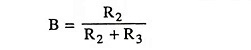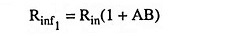## Op Amps with Negative Feedback Interview Questions and Answers:

1. What kind of negative feedback is present in a noninverting op-amp?

Ans. Negative voltage series feedback.

2. What is effect of negative feedback in a noninverting amplifier on input impedance and bandwidth?

Ans. Negative feedback in a noninverting amplifier increases each of the input impedance and bandwidth by a factor (1 + AB) where AB is feedback factor.

3. What is a voltage follower?

Ans. Voltage follower is an electronic circuit in which output voltage tracks the input voltage both in sign and magnitude.

4. What are the advantages of using a voltage follower amplifier?

Ans. Voltage follower has three unique characteristics viz extremely high input impedance, extremely low output impedance and unity transmission gain and is, therefore, an ideal circuit device for use as a buffer amplifier.

5. In what way is the voltage follower a special case of the non-inverting amplifier?

Ans. If feedback resistor is made zero or R1 is made ∞ (by keeping it open circuited) in a noninverting amplifier circuit, voltage follower is obtained.

6. What is an inverting amplifier?

Ans. In an inverting amplifier, the input is connected to the minus or inverting terminal of op-amp.

7. How does negative feedback affect the performance of an inverting amplifier?

Ans. Negative feedback decreases the voltage gain, nonlinear distortion, noise, output impedance and total output offset voltage and increases the input impedance and bandwidth.

8. What are the applications of an inverting amplifier?

Ans. Inverting amplifier is a very versatile component and can be used for performing number of mathematical stimulation such as analog inverter, paraphrase amplifier, phase shifter, adder, integrator, differentiator.

9. What is a differential amplifier?

Ans. Differential amplifier is a combination of inverting and noninverting amplifiers and amplifies the voltage difference between two input lines neither of which is grounded.

10. Are the characteristics of dual op-amp differential amplifier similar to those of inverting amplifier?

Ans. No. The characteristics of a dual op-amp differential amplifier are identical to those of a noninverting amplifier.

11. What is the drawback of dual op-amp differential amplifier?

Ans. The possible reduction in the amplitude of the output signal is the drawback of dual op-amp differential amplifier.

12. What is the effect on input resistance if two op-amps are used as differential amplifier?

Ans. Input Resistance. Input resistance of first stage, Rinf2 = Rin (1 + AB)

where Rin is the open-loop resistance of op-amp, as first stage is noninverting amplifier.With vin2 grounded i.e. vin2 = 0, the second stage also becomes a noninverting amplifier whose input resistance may be given aswhere Rin is open-loop resistance of op-amp

Scroll to Top